Author: Oscar Cronquist Article last updated on February 03, 2023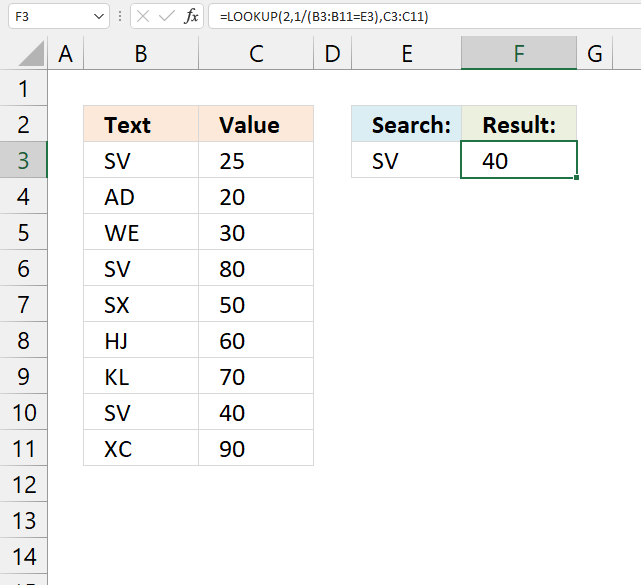This article shows a formula that performs a reverse lookup and returns the corresponding value based on the last matching value.

## 1. Find the last matching valueThe formula in cell F3 performs a lookup and matches the last item, it returns a corresponding value from column C on the same row.

For example, Item "SV" is found in cells B3, B6, and B10. The last matching value is in cell B10 and the corresponding value in column C is "40".

Formula in cell F3:

=LOOKUP(2,1/(B3:B11=E3),C3:C11)

I recommend the XLOOKUP function if you use Excel 365.

### 1.1 Explaining formula in cell F3

#### Step 1 - Compare values to search value

The equal sign compares value to value, you can also compare a single value to multiple values at the same time. That is what is going on here.

B3:B11=E3

becomes

{"SV"; "AD"; "WE"; "SV"; "SX"; "HJ"; "KL"; "SV"; "XC"}="SV"

and returns {TRUE; FALSE; FALSE; TRUE; FALSE; FALSE; FALSE; TRUE; FALSE}.

#### Step 2 - Divide 1 with result

The parentheses let you control the order of calculations, we want to perform the comparison before the division with 1.

1/(B3:B11=E3)

becomes

1/{TRUE; FALSE; FALSE; TRUE; FALSE; FALSE; FALSE; TRUE; FALSE}

and returns {1; #DIV/0!; #DIV/0!; 1; #DIV/0!; #DIV/0!; #DIV/0!; 1; #DIV/0!}.

#### Step 3 - Lookup the last matching value

The LOOKUP function allows us to match a value if it is sorted ascending, however it also lets you match the last value in an array if the others are errors.

LOOKUP(2,1/(B3:B11=E3),C3:C11)

becomes

LOOKUP(2,{1; #DIV/0!; #DIV/0!; 1; #DIV/0!; #DIV/0!; #DIV/0!; 1; #DIV/0!},C3:C11)

becomes

LOOKUP(2,{1; #DIV/0!; #DIV/0!; 1; #DIV/0!; #DIV/0!; #DIV/0!; 1; #DIV/0!}, {25; 20; 30; 80; 50; 60; 70; 40; 90})

and returns 40.

## 2. Find the last matching value - two conditions AND - logic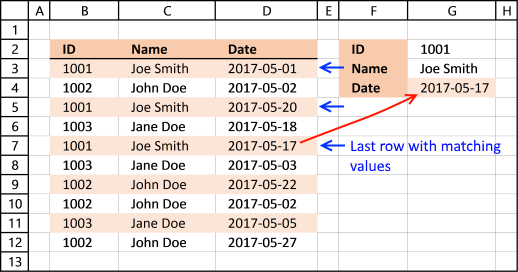### 2.1 Question

Ok, you've shown it for regular ranges....how about within tables.I have a table similar to:ID Name Date
1001 Joe Smith 5/1/2017
1002 John Doe 5/2/2017
1001 Joe Smith 5/17/2017
1003 Jane Doe 5/18/2017
1001 Joe Smith 5/20/2017

### 2.2 Formula

The formula below lets you search for criteria and return the last matching record in the table.

Example, 1001 and Joe Smith is found on row 3,5 and 7. The record on row 7 is the last record in the table so the formula returns the date (2017-05-17) from row 7, in cell G4.

Formula in cell G4:

=LOOKUP(2,1/((B3:B12=G2)*(C3:C12=G3)),D3:D12)

This formula is weird, you don't need to enter it as an array formula as you should. I don't know why, however this gave me an idea, check it out here:
Create a list of unique distinct values

Recommended article

Recommended articles

Find last matching value in an unsorted list
This article demonstrates a formula that returns the last matching value based on a given condition. The above image shows […]

### 2.3 Explaining formula in cell G4

#### Step 1 - Construct first logical expression (ID)

We want to find all to find values in column B equal to 1001. The equal sign lets you compare value to values, not case sensitive.

B3:B12=G2

becomes

{1001; 1002; 1001; 1003; 1001; 1003; 1002; 1002; 1003; 1002}=1001

and returns

{TRUE; FALSE; TRUE; FALSE; TRUE; FALSE; FALSE; FALSE; FALSE; FALSE}

#### Step 2 - Construct second logical expression (NAME)

The second logical expressions checks if values in cell range C3:C12 is equal to "Joe Smith"

C3:C12=G3

becomes

{"Joe Smith"; "John Doe"; "Joe Smith"; "Jane Doe"; "Joe Smith"; "Jane Doe"; "John Doe"; "John Doe"; "Jane Doe"; "John Doe"}="Joe Smith"

and returns

{TRUE; FALSE; TRUE; FALSE; TRUE; FALSE; FALSE; FALSE; FALSE; FALSE}

#### Step 3 - Multiply arrays

Both conditions must be met so we must multiply (*) the arrays. If we wanted at least one of two conditions met we would add the arrays (+)

(B3:B12=G2)*(C3:C12=G3)

becomes

{TRUE; FALSE; TRUE; FALSE; TRUE; FALSE; FALSE; FALSE; FALSE; FALSE} * {TRUE; FALSE; TRUE; FALSE; TRUE; FALSE; FALSE; FALSE; FALSE; FALSE}

and returns

{1; 0; 1; 0; 1; 0; 0; 0; 0; 0}

#### Step 4 - Dividing by zero returns an error

The LOOKUP function allows us to match a value if it is sorted ascending, however it also lets you match the last value in an array if the others are errors.

1/((B3:B12=G2)*(C3:C12=G3))

becomes

1/{1;0;1;0;1;0;0;0;0;0}

and returns

{1;#DIV/0!;1;#DIV/0!; 1;#DIV/0!; #DIV/0!; #DIV/0!;#DIV/0!; #DIV/0!}

1/0 returns #DIV/0!

#### Step 5 - Find the last value in the array

The LOOKUP function finds the last value in the array and returns the corresponding value on the same row in cell range D3:D12.

LOOKUP(2,1/((B3:B12=G2)*(C3:C12=G3)),D3:D12)

becomes

LOOKUP(2,{1;#DIV/0!;1;#DIV/0!; 1;#DIV/0!; #DIV/0!; #DIV/0!;#DIV/0!; #DIV/0!},D3:D12)

becomes

LOOKUP(2,{1; #DIV/0!; 1; #DIV/0!; 1; #DIV/0!; #DIV/0!; #DIV/0!; #DIV/0!; #DIV/0!},{42856; 42857; 42875; 42873; 42872; 42858; 42877; 42857; 42860; 42882})

and returns  2017-05-17 (42872)  in cell G4.

Recommended articles

How to use the LOOKUP function
Finds a value in a sorted cell range and returns a value on the same row.

## 3. Find the last matching value - two conditions OR - logic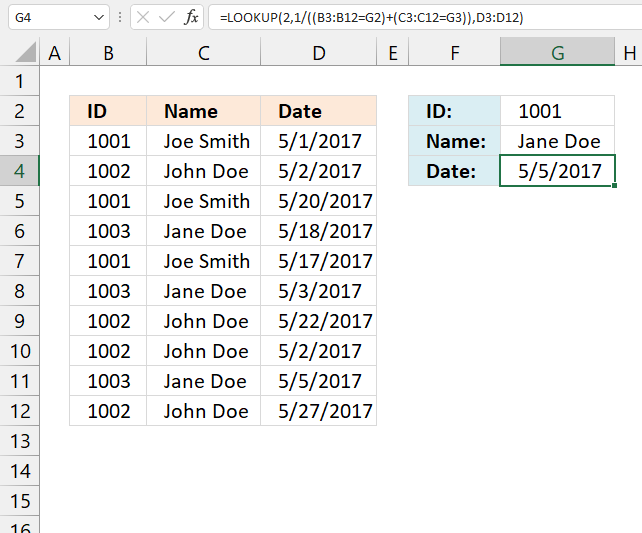The image above demonstrates a formula in cell G4 that returns a value if at least one of two possible conditions match on the same row, however, the formula performs a reverse lookup meaning it starts with the last value and moves up. As soon as any of the two conditions match the corresponding value from column D is returned.

For example, the first condition is specified in cell G2 and the second is specified in cell G3. The formula starts with the last row (12) and goes up. "Jane Doe" is the first match in cell C11, the formula returns the corresponding value "5/5/2017" from column D on the same row (11).

Formula in cell G4:

=LOOKUP(2, 1/((B3:B12=G2)+(C3:C12=G3)), D3:D12)

### 3.1 Explaining formula in cell G4

#### Step 1 - Compare first lookup value to values

The equal sign is a logical operator meaning it lets you compare value to values, not case sensitive. The result is a logical value or boolean value, in this case, multiple values.

B3:B12=G2

becomes

{1001; 1002; 1001; 1003; 1001; 1003; 1002; 1002; 1003; 1002}= 1001

and returns

{TRUE; FALSE; TRUE; FALSE; TRUE; FALSE; FALSE; FALSE; FALSE; FALSE}.

#### Step 2 - Compare second lookup value to values

C3:C12=G3

becomes

{"Joe Smith"; "John Doe"; "Joe Smith"; "Jane Doe"; "Joe Smith"; "Jane Doe"; "John Doe"; "John Doe"; "Jane Doe"; "John Doe"}="Jane Doe"

and returns

{FALSE; FALSE; FALSE; TRUE; FALSE; TRUE; FALSE; FALSE; TRUE; FALSE}.

#### Step 3 - Add array values

The plus sign adds the arrays containing boolean values.

(B3:B12=G2)+(C3:C12=G3)

becomes

{TRUE; FALSE; TRUE; FALSE; TRUE; FALSE; FALSE; FALSE; FALSE; FALSE} + {FALSE; FALSE; FALSE; TRUE; FALSE; TRUE; FALSE; FALSE; TRUE; FALSE}

and returns

{1; 0; 1; 1; 1; 1; 0; 0; 1; 0}.

#### Step 4 - Divide 1 with array

We want to create an error value if the array contains a 0 (zero). The LOOKUP function ignores error values.

1/((B3:B12=G2)+(C3:C12=G3))

becomes

1/{1; 0; 1; 1; 1; 1; 0; 0; 1; 0}

and returns {1; #DIV/0!; 1; 1; 1; 1; #DIV/0!; #DIV/0!; 1; #DIV/0!}.

#### Step 5 - Find a match and return the corresponding value

The LOOKUP function allows us to match a value if it is sorted ascending, however it also lets you match the last value in an array if the others are errors.

LOOKUP(2, 1/((B3:B12=G2)+(C3:C12=G3)), D3:D12)

becomes

LOOKUP(2, {1; #DIV/0!; 1; 1; 1; 1; #DIV/0!; #DIV/0!; 1; #DIV/0!}, D3:D12)

becomes

LOOKUP(2, {1; #DIV/0!; 1; 1; 1; 1; #DIV/0!; #DIV/0!; 1; #DIV/0!}, {42856; 42857; 42875; 42873; 42872; 42858; 42877; 42857; 42860; 42882})

and returns 42860 (5/5/2017).

## 4. Find the last matching value based on a list of values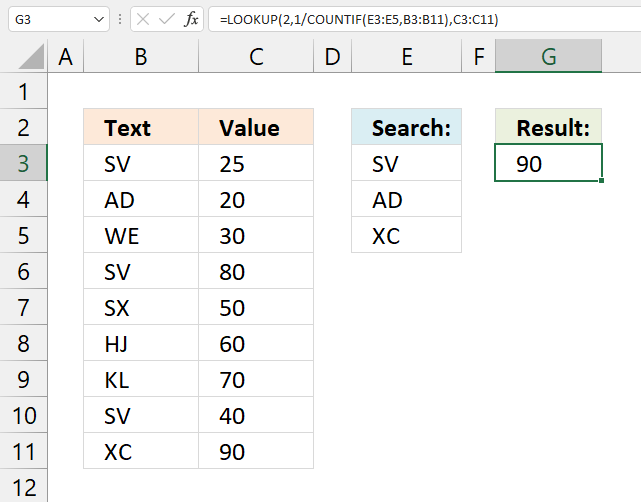The formula in cell G3 shown in the image above performs a reverse lookup using multiple values specified in cell range E3:E5. It starts with the last value which is cell B11 and checks if any of the values match.

The last lookup value "XC" matches cell B11 so the formula returns the corresponding value from column C on the same row which is "90".

Formula in cell G3:

=LOOKUP(2, 1/COUNTIF(E3:E5, B3:B11), C3:C11)

### 4.1 Explaining formula in cell G3

#### Step 1 - Count values based on list

The COUNTIF function counts values matching any value in cell range E3:E5.

COUNTIF(E3:E5, B3:B11)

becomes

COUNTIF({"SV"; "AD"; "XC"}, {"SV"; "AD"; "WE"; "SV"; "SX"; "HJ"; "KL"; "SV"; "XC"})

and returns {1; 1; 0; 1; 0; 0; 0; 1; 1}.

#### Step 2 - Divide 1 with array

We want to create an error value if the array contains a 0 (zero). The LOOKUP function ignores error values which will be demonstrated in the next step.

1/COUNTIF(E3:E5, B3:B11)

becomes

1/{1; 1; 0; 1; 0; 0; 0; 1; 1}

and returns

{1; 1; #DIV/0!; 1; #DIV/0!; #DIV/0!; #DIV/0!; 1; 1}.

#### Step 3 - Lookup value and return corresponding value

The LOOKUP function allows you to match the last value in an array (reverse lookup) if the other values are errors.

LOOKUP(2, 1/COUNTIF(E3:E5, B3:B11), C3:C11)

becomes

LOOKUP(2, {1; 1; #DIV/0!; 1; #DIV/0!; #DIV/0!; #DIV/0!; 1; 1}, C3:C11)

becomes

LOOKUP(2, {1; 1; #DIV/0!; 1; #DIV/0!; #DIV/0!; #DIV/0!; 1; 1}, {25; 20; 30; 80; 50; 60; 70; 40; 90})

and returns 90 in cell G3.

## 5. Find the last matching date based on a date range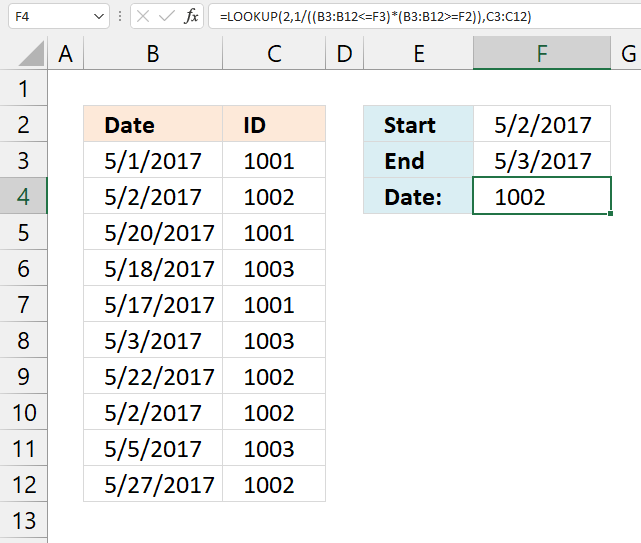The image above demonstrates a formula in cell F4 that uses a date range specified in cell F2 and F3 to perform a reverse lookup using all dates in the date range against dates in cell range B3:B12.

For example, the formula begins with the last date which is in cell B12, and moves up. There is not a match until cell B10 which contains the date "5/2/2017", that date is in the date range specified in cells F2:F3.

The corresponding value from the same row in column C is "1002", that value is returned in cell F4.

Formula in cell F4:

=LOOKUP(2, 1/((C3:C12<=G3)*(C3:C12>=G2)), D3:D12)

### 5.1 Explaining formula in cell F4

#### Step 1 - Compare end date to dates

The less than and equal sign combined checks if the dates are equal or smaller than the end date.

C3:C12<=G3

becomes

{42856; 42857; 42875; 42873; 42872; 42858; 42877; 42857; 42860; 42882}<=42858

and returns

{TRUE; TRUE; FALSE; FALSE; FALSE; TRUE; FALSE; TRUE; FALSE; FALSE}.

#### Step 2 - Compare start date to dates

The larger than and equal sign combined checks if the dates are equal or larger than the start date.

C3:C12>=G2

becomes

{42856; 42857; 42875; 42873; 42872; 42858; 42877; 42857; 42860; 42882}>=42857

and returns

{FALSE; TRUE; TRUE; TRUE; TRUE; TRUE; TRUE; TRUE; TRUE; TRUE}.

#### Step 3 - Multiply arrays

The asterisk character lets you multiply the arrays meaning you apply AND logic. AND logic works like this: TRUE*TRUE = TRUE (1) , TRUE * FALSE = FALSE (0)

When you perform a calculation in Excel boolean values are converted to their numerical equivalents. TRUE = 1 and FALSE = 0 (zero)

(C3:C12<=G3)*(C3:C12>=G2)

becomes

{TRUE; TRUE; FALSE; FALSE; FALSE; TRUE; FALSE; TRUE; FALSE; FALSE} * {FALSE; TRUE; TRUE; TRUE; TRUE; TRUE; TRUE; TRUE; TRUE; TRUE}

and returns {0; 1; 0; 0; 0; 1; 0; 1; 0; 0}.

#### Step 4 - Divide 1 with array

1/((C3:C12<=G3)*(C3:C12>=G2))

becomes

1/{0; 1; 0; 0; 0; 1; 0; 1; 0; 0}

and returns {#DIV/0!; 1; #DIV/0!; #DIV/0!; #DIV/0!; 1; #DIV/0!; 1; #DIV/0!; #DIV/0!}.

#### Step 5 - Reverse lookup based on date range

LOOKUP(2, 1/((C3:C12<=G3)*(C3:C12>=G2)), D3:D12)

becomes

LOOKUP(2,{#DIV/0!; 1; #DIV/0!; #DIV/0!; #DIV/0!; 1; #DIV/0!; 1; #DIV/0!; #DIV/0!}, D3:D12)

becomes

LOOKUP(2,{#DIV/0!; 1; #DIV/0!; #DIV/0!; #DIV/0!; 1; #DIV/0!; 1; #DIV/0!; #DIV/0!},{1001; 1002; 1001; 1003; 1001; 1003; 1002; 1002; 1003; 1002})

and returns 1002.

## 6. Find the last matching week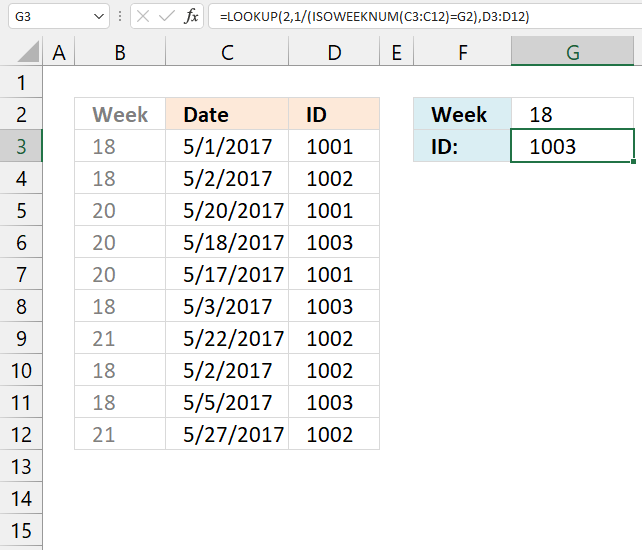The image above shows a formula in cell G3 that performs a reverse lookup using a week number specified in cell G2.

It returns a value from column D if the corresponding cell in column C matches the lookup value.

Formula in cell G3:

=LOOKUP(2, 1/(ISOWEEKNUM(C3:C12)=G2), D3:D12)

### 6.1 Explaining formula in cell G3

#### Step 1 - Convert dates to week numbers

The ISOWEEKNUM function calculates a number based on the ISO week number of the year for a given date.

ISOWEEKNUM(C3:C12)

becomes

ISOWEEKNUM({42856; 42857; 42875; 42873; 42872; 42858; 42877; 42857; 42860; 42882})

and returns {18; 18; 20; 20; 20; 18; 21; 18; 18; 21}.

#### Step 2 - Compare week numbers to the condition

ISOWEEKNUM(C3:C12)=G2

becomes

{18; 18; 20; 20; 20; 18; 21; 18; 18; 21}=18

and returns {TRUE; TRUE; FALSE; FALSE; FALSE; TRUE; FALSE; TRUE; TRUE; FALSE}.

#### Step 3 - Divide 1 with array

1/(ISOWEEKNUM(C3:C12)=G2)

becomes

1/{TRUE; TRUE; FALSE; FALSE; FALSE; TRUE; FALSE; TRUE; TRUE; FALSE}

and returns {1; 1; #DIV/0!; #DIV/0!; #DIV/0!; 1; #DIV/0!; 1; 1; #DIV/0!}.

#### Step 4 - Reverse lookup based on week number

LOOKUP(2, 1/(ISOWEEKNUM(C3:C12)=G2), D3:D12)

becomes

LOOKUP(2, {1; 1; #DIV/0!; #DIV/0!; #DIV/0!; 1; #DIV/0!; 1; 1; #DIV/0!}, D3:D12)

becomes

LOOKUP(2,{1; 1; #DIV/0!; #DIV/0!; #DIV/0!; 1; #DIV/0!; 1; 1; #DIV/0!},{1001; 1002; 1001; 1003; 1001; 1003; 1002; 1002; 1003; 1002})

and returns 1003.

## 7. Find the last matching month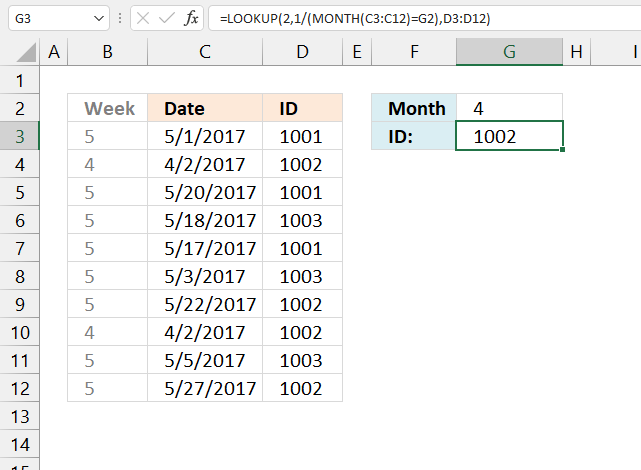The picture above demonstrates a formula in cell G3 that performs a reverse lookup using a month number specified in cell G2.

It returns a value from column D if the corresponding cell in column C matches the lookup value.

For example, column B contains numbers representing the relative position of a month. 1 is January, 2 is February ... 12 is December.

Cell G2 contains 4, the formula starts with the last cell in column C and goes up. Cell C10 contains a date in April which is month number 4, it matches number 4 in cell G2.

The corresponding value in column D on the same row is returned to cell G3.

Formula in cell G3:

=LOOKUP(2,1/(MONTH(C3:C12)=G2), D3:D12)

### 7.1 Explaining formula in cell G3

#### Step 1 - Calculate number representing the month

The MONTH function calculates the month as a number from an Excel date.

MONTH(C3:C12)

becomes

MONTH({42856; 42827; 42875; 42873; 42872; 42858; 42877; 42827; 42860; 42882})

and returns {5; 4; 5; 5; 5; 5; 5; 4; 5; 5}.

#### Step 2 - Compare month numbers to lookup value

MONTH(C3:C12)=G2

becomes

{5; 4; 5; 5; 5; 5; 5; 4; 5; 5}=4

and returns {FALSE; TRUE; FALSE; FALSE; FALSE; FALSE; FALSE; TRUE; FALSE; FALSE}.

#### Step 3 - Divide 1 with array

1/(MONTH(C3:C12)=G2)

becomes

1/{FALSE; TRUE; FALSE; FALSE; FALSE; FALSE; FALSE; TRUE; FALSE; FALSE}

and returns {#DIV/0!; 1; #DIV/0!; #DIV/0!; #DIV/0!; #DIV/0!; #DIV/0!; 1; #DIV/0!; #DIV/0!}.

#### Step 4 - Reverse lookup based on month number

LOOKUP(2, 1/(MONTH(C3:C12)=G2), D3:D12)

becomes

LOOKUP(2, {#DIV/0!; 1; #DIV/0!; #DIV/0!; #DIV/0!; #DIV/0!; #DIV/0!; 1; #DIV/0!; #DIV/0!}, D3:D12)

becomes

LOOKUP(2, {#DIV/0!; 1; #DIV/0!; #DIV/0!; #DIV/0!; #DIV/0!; #DIV/0!; 1; #DIV/0!; #DIV/0!}, {1001; 1002; 1001; 1003; 1001; 1003; 1002; 1002; 1003; 1002})

and returns 1002.

##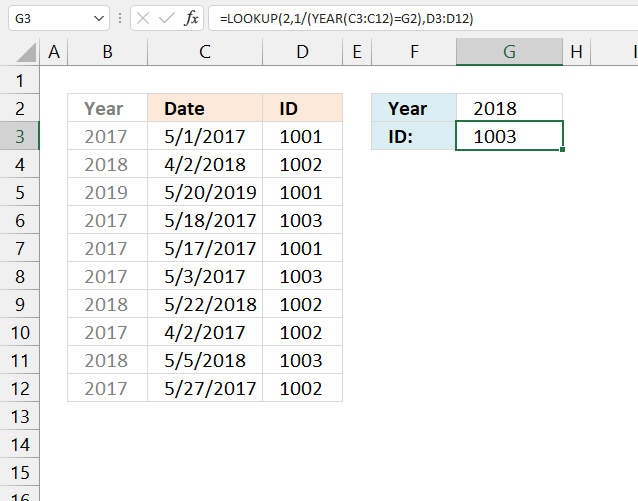The formula in cell G3 performs a reverse lookup using the year number in cell G2 against dates in column C.

The last match is found in cell C11 and the corresponding value in column D on the same row is returned to cell G3.

Formula in cell G3:

=LOOKUP(2,1/(YEAR(C3:C12)=G2),D3:D12)

### 8.1 Explaining formula in cell G3

#### Step 1 - Calculate year

The YEAR function extracts the year from an Excel date.

YEAR(C3:C12)

becomes

YEAR({42856; 43192; 43605; 42873; 42872; 42858; 43242; 42827; 43225; 42882})

and returns {2017; 2018; 2019; 2017; 2017; 2017; 2018; 2017; 2018; 2017}.

#### Step 2 - Compare year numbers to lookup value

YEAR(C3:C12)=G2

becomes

{2017; 2018; 2019; 2017; 2017; 2017; 2018; 2017; 2018; 2017}=2018

and returns

{FALSE; TRUE; FALSE; FALSE; FALSE; FALSE; TRUE; FALSE; TRUE; FALSE}

#### Step 3 - Divide 1 with array

1/(YEAR(C3:C12)=G2)

becomes

1/{FALSE; TRUE; FALSE; FALSE; FALSE; FALSE; TRUE; FALSE; TRUE; FALSE}

and returns {#DIV/0!; 1; #DIV/0!; #DIV/0!; #DIV/0!; #DIV/0!; 1; #DIV/0!; 1; #DIV/0!}.

#### Step 4 - Lookup year and return value based on last match

LOOKUP(2, 1/(YEAR(C3:C12)=G2), D3:D12)

becomes

LOOKUP(2, {#DIV/0!; 1; #DIV/0!; #DIV/0!; #DIV/0!; #DIV/0!; 1; #DIV/0!; 1; #DIV/0!}, {1001; 1002; 1001; 1003; 1001; 1003; 1002; 1002; 1003; 1002})

and returns 1003 in cell G3.